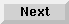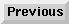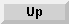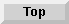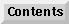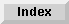5 Operators and Functions

## Logical Operators

The logical operators (sometimes called Boolean operators) are AND, OR, EXOR and NOT. These operators are most frequently used as part of an IFTHEN statement, as shown in the next chapter.

AND compares two expressions. If both expressions are true (a non-zero value), the result is true. If one or both of the expressions are false (zero), the result is false.

numeric expression AND numeric expression

OR (inclusive OR) compares two expressions. If either expression is true, the result is true. If both expressions are true, the result is true. If both expressions are false, the result is false.

numeric expression OR numeric expression

EXOR (exclusive OR) compares two expressions. If only one of the expressions is true, the result is true. If both are true or both are false, the result is false.

numeric expression EXOR numeric expression

NOT changes the logical value of an expression. If the expression is true, NOT changes it to false. If the expression is false, NOT changes it to true.

numeric expression EXOR numeric expression

As in the case of relational operators, if the result is true, a 1 is returned; if the result is false, a 0 is returned.

The expressions that the logical operators compare can be either relational or non-relational:

• If the expression is relational , its true or false designation is determined by the relational operation.

• If the expression is non-relational (A), it is true if its arithmetic value is not equal to 0; it is false if its arithmetic value equals 0.

For the following examples, assume A=0, B=2, C=4, and D=4:

A < B AND C = D
True. Both relational expressions A<B and C=D are true.
A AND C = D
False. The arithmetic value of A equals 0 (false).
A OR B
True. The arithmetic value of B is not 0 (so B is true).
A EXOR B
True. One value is true and one value is false.
NOT A
True. A is 0 (false).
NOT B OR NOT C
False. NOT B is false and NOT C is false.
A truth table can be used to summarize logical operations:

Columns
A B A AND B A OR B A EXOR B NOT A
TT1100
TF0110
FT0111
FF0001

Eloquence Language Manual - 19 DEC 2002